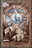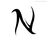Button for Adding Records to Subtable with Numerical IncreaseI have a parent table named Apps Projects KSW and subtable named Trial Summary.

I have a button in Apps Projects KSW that adds new records to the subtable. I want those records to increase numerically in a column named Trial Number. To do this I know I need to reference the Trial Number column, but only within that specific iteration of the subtable. Otherwise the trial numbers continue where the previous subtable left off. I want to be able to have trials numbered 1 through 5 (or more) in every iteration of the subtable without having to manually enter the numbers. Is it possible to accomplish this by modifying the button code, or do I need to have additional formulas elsewhere?

Here's the button code I have to create a new record only (no numercial increase):

`let myID := this;`
`let newRec := (create 'Trial Summary');`
`newRec.('Apps Projects KSW' := myID)`

I'm hoping it's an easy fix as I have more subtables that will require a similar solution.Ι'm using this method:

Create a formula field i.e. 'Item No' to the Trial Summary table with this code

let myID := number(Id);
let myTrialSum := 'Trial Summary';
if 'Trial Summary' then
cnt((select 'Apps Projects KSW')['Trial Summary' = myTrialSum and number(Id) <= myID])
else
0
end